Courses
Courses for Kids
Free study material
Free LIVE classes
More

# NCERT Solutions for Class 9 Maths Chapter 11 - ExerciseLIVE
Join Vedantu’s FREE Mastercalss

## NCERT Solutions for Class 9 Maths Chapter 11 Constructions (Ex 11.1) Exercise 11.1

Free PDF download of NCERT Solutions for Class 9 Maths Chapter 11 Exercise 11.1 (Ex 11.1) and all chapter exercises at one place prepared by expert teacher as per NCERT (CBSE) books guidelines. Class 9 Maths Chapter 11 Constructions Exercise 11.1 Questions with Solutions to help you to revise complete Syllabus and Score More marks. Register and get all exercise solutions in your emails. Download Maths NCERT Solutions Class 9 at Vedantu. Students can also avail of NCERT Solutions Class 9 Science from our website. Besides, find NCERT Book Solutions to get more understanding of various subjects.

### List of Topics Covered Under NCERT Solutions for Class 9 Maths Chapter 11

 Introduction Basic Construction Some Constructions of Triangles
Last updated date: 30th Sep 2023
Total views: 514.5k
Views today: 9.14k

## Access NCERT Solutions for Class-9 Maths Chapter 11 – Construction

Exercise (11.1)

1. Construct an angle of $\mathbf{90}{}^\circ$ at the initial point of a given ray and justify the construction.

Ans: The below given steps will be followed to construct an angle of$90{}^\circ$.

(i) Take the given ray $PQ$. Draw an arc of some radius taking point $P$ as its centre, which intersects $PQ$ at $R$.

(ii) Taking $R$ as a centre and with the same radius as before, draw an arc intersecting the previously drawn arc at $S$.

(iii) Taking $S$ as a centre and with the same radius as before, draw an arc intersecting the arc at $T$(see figure).

(iv) Taking $S$ and $T$ as the centre, draw an arc of the same radius to intersect each other at $U$.

(v) Join $PU$, which is the required ray making $90{}^\circ$ with the given ray $PQ$.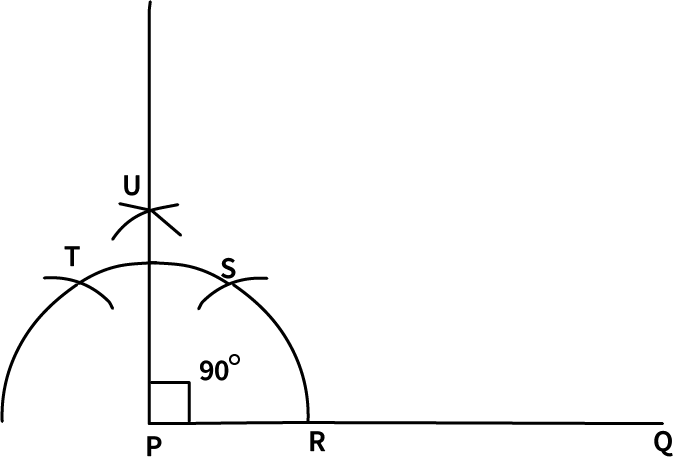Justification

We can justify the construction, if we can prove $\angle UPQ=90{}^\circ .$ For this, join $~PS$ and $PT.$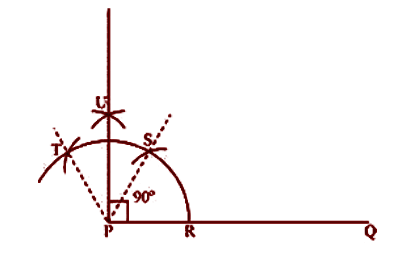We have, $\angle SPQ=\angle TPS=60{}^\circ .$In (iii) and (iv) steps of this

construction, $PU$was drawn as the bisector of $\angle TPS.$

$\angle UPS=\frac{1}{2}\angle TPS$

$=\frac{1}{2}\times {{60}^{o}}$

$={{30}^{o}}$

Also,

$\angle UPQ=\angle SPQ+\angle UPS$

$={{60}^{o}}+{{30}^{o}}$

$={{90}^{o}}$

2. Construct an angle of $\mathbf{45}{}^\circ$at the initial point of a given ray and justify the construction.

Ans: The below given steps will be followed to construct an angle of $45{}^\circ$.

Take the given ray $PQ$. Draw an arc of some radius taking point $P$as its centre, which intersects $PQ$ at $R$.

Taking $R$ as centre and with the same radius as before, draw an arc intersecting the previously drawn arc at $S$.

Taking $S$ as a centre and with the same radius as before, draw an arc intersecting the arc at $T$ (see figure).

Taking $S$ and $T$ as the centre, draw an arc of the same radius to intersect each other at $U$.

Join $PU$. Let it intersect the arc at point $V$.

From $R$ and $V$, draw arcs with radius more than $RV$to intersect each other at $W$. Join $PW$. $PW$ is the required ray making $45{}^\circ$with $PQ.$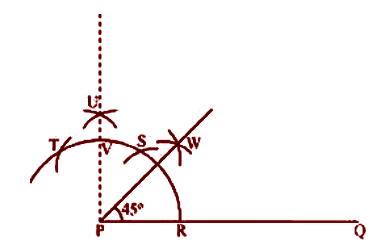Justification of Construction:

We can justify the construction if we can prove ∠WPQ = 45°. For this, join PS and PT.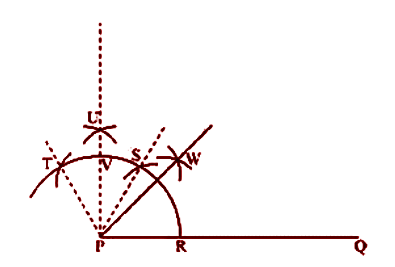We have,$\angle SPQ=\angle TPS=60{}^\circ$. In (iii) and (iv) steps of this construction, $PU$was drawn as the bisector of $\angle TPS.$

$\angle UPS=\frac{1}{2}\angle TPS$

$=\frac{1}{2}\times {{60}^{o}}$

$={{30}^{o}}$

Also,

$\angle UPQ=\angle SPQ+\angle UPS$

$={{60}^{o}}+{{30}^{o}}$

$={{90}^{o}}$

In step (vi) of this construction, PW was constructed as the bisector of ∠UPQ.

$\angle WPQ=\frac{1}{2}\angle UPQ$

$=\frac{1}{2}\times {{90}^{o}}$

$={{45}^{o}}$

3. Construct the angles of the following measuremet

I. $30{}^\circ$

Ans: The below given steps will be followed to construct an angle of $30{}^\circ$.

(1) Draw the given ray $PQ$. Taking $P$ as a centre and with some radius, draw an arc of a circle which intersects $PQ$ at $R$.

(2) Taking $R$ as centre and with the same radius as before, draw an arc intersecting the previously drawn arc at point $S$.

(3) Taking $R$ and $S$ as centre and with radius more than $RS$, draw arcs to intersect each other at $T$. Join $PT$which is the required ray making $30{}^\circ$ with the given ray $PQ$.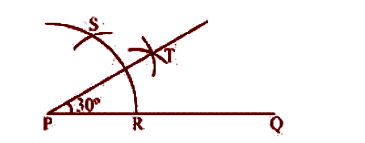II. ${{22}^{o}}\frac{1}{2}$

Ans: The below given steps will be followed to construct an angle of ${{22}^{o}}\frac{1}{2}$.

Take the given ray $PQ$. Draw an arc of some radius, taking point $P$ as its centre, which intersects $PQ$ at $R$.

Taking $R$ as centre and with the same radius as before, draw an arc intersecting the previously drawn arc at $S$.

Taking $S$ as centre and with the same radius as before, draw an arc intersecting the arc at $T$ (see figure).

Taking $S$ and $T$ as centre, draw an arc of same radius to intersect each other at $U$.

Join$PU$. Let it intersect the arc at point $V$.

From $R$ and $V$, draw arcs with radius more than $RV$ to intersect each other at $W$. Join $PW$.

Let it intersect the arc at $X$. Taking $X$ and $R$as centre and radius more than $RX$, draw arcs to intersect each other at $Y$.

Joint $PY$ which is the required ray making ${{22}^{o}}\frac{1}{2}$ with the given ray $PQ$.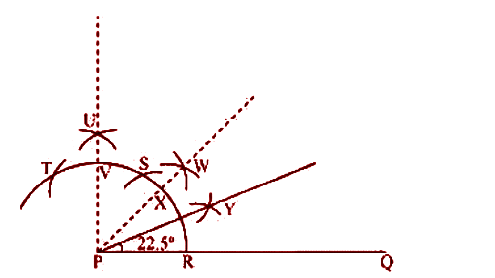III. $15{}^\circ$

Ans: The below given steps will be followed to construct an angle of $15{}^\circ$.

(1) Draw the given ray $PQ$. Taking $P$ as centre and with some radius, draw an arc of a circle which intersects $PQ$ at $R$.

(2) Taking $R$ as centre and with the same radius as before, draw an arc intersecting the previously drawn arc at point $S$.

(3) Taking $R$ and $S$ as centre and with radius more than $RS$, draw arcs to intersect each other at $T$. Join $PT$.

(4) Let it intersect the arc at $U$. Taking $U$ and $R$ as centre and with radius more than $RU$, draw an arc to intersect each other at $V$. Join $PV$ which is the required ray making $15{}^\circ$ with the given ray $PQ$.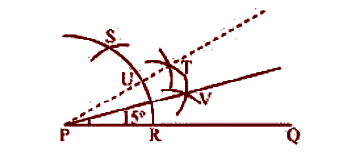4. Construct the following angles and verify by measuring them by a protractor:

(i) $75{}^\circ$

Ans: The below given steps will be followed to construct an angle of $75{}^\circ$.

Take the given ray $PQ$. Draw an arc of some radius taking point $P$ as its centre, which intersects $PQ$ at $R$.

Taking $R$ as centre and with the same radius as before, draw an arc intersecting the previously drawn arc at $S$.

Taking $S$ as centre and with the same radius as before, draw an arc intersecting the arc at $T$ (see figure).

Taking $S$ and $T$ as centre, draw an arc of same radius to intersect each other at $U$.

Join $PU$. Let it intersect the arc at $V$. Taking $S$ and $V$ as centre, draw arcs with radius more than $SV$. Let those intersect each other at $W$. Join $PW$ which is the required ray making $75{}^\circ$ with the given ray $PQ$ .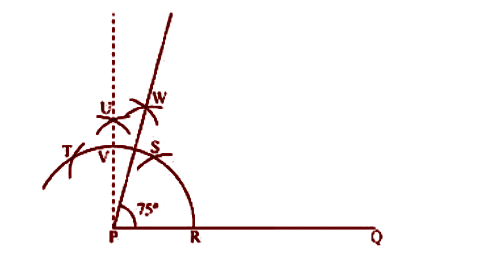The angle so formed can be measured with the help of a protractor. It comes to be $75{}^\circ$.

(ii) $105{}^\circ$

Ans: The below given steps will be followed to construct an angle of $105{}^\circ$

Take the given ray $PQ$. Draw an arc of some radius taking point $P$ as its centre, which intersects $PQ$ at $R$.

Taking $R$ as centre and with the same radius as before, draw an arc intersecting the previously drawn arc at $S$.

Taking $S$ as centre and with the same radius as before, draw an arc intersecting the arc at $T$ (see figure).

Taking $S$ and $T$as centre, draw an arc of same radius to intersect each other at U.

Join $PU$. Let it intersect the arc at $V$. Taking $T$ and $V$ as centre, draw arcs with radius more than $TV$. Let these arcs intersect each other at W. Join $PW$ which is the required ray making $105{}^\circ$ with the given ray $PQ$.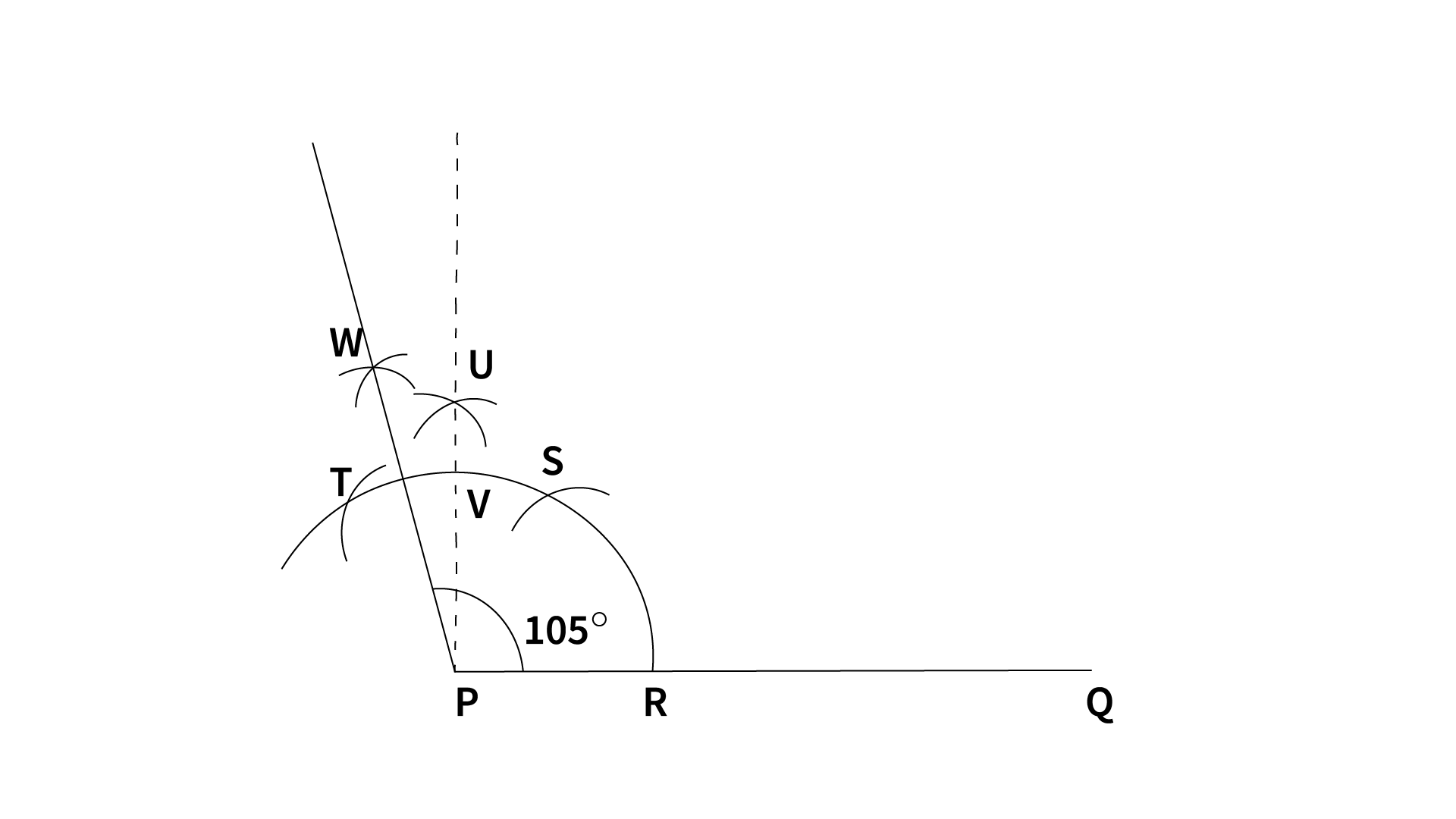The angle so formed can be measured with the help of a protractor. It comes to be $105{}^\circ$

(iii) $135{}^\circ$

Ans: The below given steps will be followed to construct an angle of $135{}^\circ$.

(1) Take the given ray $PQ$. Extend $PQ$ on the opposite side of $Q$. Draw a semi-circle of some radius taking point P as its centre, which intersects $PQ$ at $R$and$W$.

(2) Taking $R$ as centre and with the same radius as before, draw an arc intersecting the previously drawn arc at $S$.

(3) Taking $S$ as centre and with the same radius as before, draw an arc intersecting the arc at $T$ (see figure).

(4) Taking $S$ and $T$ as centre, draw an arc of same radius to intersect each other at U.

(5) Join $PU$. Let it intersect the arc at $V$. Taking $V$and $W$as centre and with radius more than $\frac{1}{2}TV$, draw arcs to intersect each other at $X$. Join $PX,$which is the required ray making $135{}^\circ$ with the given line $PQ$.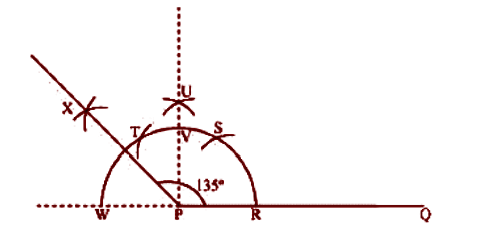The angle so formed can be measured with the help of a protractor. It comes to be $135{}^\circ$.

5. Construct an equilateral triangle, given its side and justify the construction.

Ans: Let us draw an equilateral triangle of side $5cm$. We know that all sides of an equilateral triangle are equal. Therefore, all sides of the equilateral triangle will be $5cm$. We also know that each angle of an equilateral triangle is$60{}^\text{o}$. The below given steps will be followed to draw an equilateral triangle of $5cm$ side.

Step I: Draw a line segment $AB$of $5cm$ length. Draw an arc of some radius, while taking $A$ as its centre. Let it intersect $AB$ at $P$.

Step II: Taking $P$ as centre, draw an arc to intersect the previous arc at $E$. Join $AE$.

Step III: Taking $A$ as centre, draw an arc of $5cm$ radius, which intersects extended line segment $AE$ at $C$. Join $AC$ and $BC$. $\Delta ABC$is the required equilateral triangle of side $5cm$.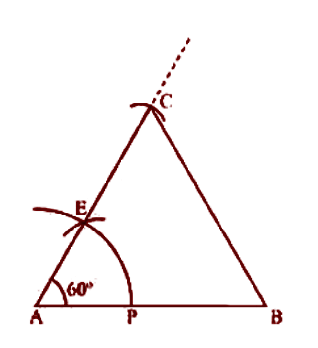Justification of Construction:

We can justify the construction by showing $ABC$as an equilateral triangle i.e.,

$AB\text{ }=\text{ }BC\text{ }=\text{ }AC\text{ }=\text{ }5cm$

and $\angle A\text{ }=\angle B\text{ }=\angle C\text{ }=\text{ }60{}^\circ .$

In $\Delta ABC$, we have $AB\text{ }=\text{ }BC\text{ }=\text{ }AC\text{ }=\text{ }5cm$and $\angle A\text{ }=\text{ }60{}^\circ$.

Since $AC\text{ }=\text{ }AB,\angle B\text{ }=\angle C$(Angles opposite to equal sides of a triangle)

In $\Delta ABC$,

$\angle A\text{ }+\angle B\text{ }+\angle C\text{ }=\text{ }180{}^\circ$ (Angle sum property of a triangle)

$~\Rightarrow 60{}^\circ \text{ }+\angle C\text{ }+\angle C\text{ }=\text{ }180{}^\circ$

$\Rightarrow 60{}^\circ \text{ }+\text{ }2\angle C\text{ }=\text{ }180{}^\circ$

$\Rightarrow 2\angle C\text{ }=\text{ }180{}^\circ \text{ }-\text{ }60{}^\circ \text{ }=\text{ }120{}^\circ$

$\Rightarrow \angle C\text{ }=\text{ }60{}^\circ$

$\Rightarrow \angle B\text{ }=\angle C\text{ }=\text{ }60{}^\circ$

We have,$\angle A\text{ }=\angle B\text{ }=\angle C\text{ }=\text{ }60{}^\circ$... (1)

$\angle A\text{ }=\angle B$and $\angle A\text{ }=\angle C$

$BC\text{ }=\text{ }AC$and $BC\text{ }=\text{ }AB$(Sides opposite to equal angles of a triangle)

$AB\text{ }=\text{ }BC\text{ }=\text{ }AC\text{ }=\text{ }5\text{ }cm$... (2)

From Equations (1) and (2), $\Delta ABC$is an equilateral triangle

### A Glance about the Topics

From the Chapter 11 Construction topics, students can learn how to bisect the straight line or make certain angles using a compass and ruler.

• To bisect a straight line at 90°, take a compass and make an arc from each end of a line by taking a measurement of more than half of the line. Join the arcs to get the bisector of a straight line.

• To bisect an angle of the triangle, ΔABC, draw an arc that intersects two lines AB and BC. Measure the two points that cut the two lines AB and BC and draw an arc, by keeping two points as the centre. Now join the arc with point B to get a bisector of an angle.

NCERT Solutions for Class 9 Maths

### NCERT Solution Class 9 Maths of Chapter 11 All Exercises

 Chapter 11 - Constructions Exercises in PDF Format Exercise 11.1 5 Questions & Solutions (2 Short Answers, 2 Long Answer, 1 Very Long Answer) Exercise 11.2 5 Questions & Solutions (5 Very Long Answers)

## NCERT Solutions for Class 9 Maths Chapter 11 Constructions Exercise 11.1

Opting for the NCERT solutions for Ex 11.1 Class 9 Maths is considered as the best option for the CBSE students when it comes to exam preparation. This chapter consists of many exercises. Out of which we have provided the Exercise 11.1 Class 9 Maths NCERT solutions on this page in PDF format. You can download this solution as per your convenience or you can study it directly from our website/ app online.

Vedantu in-house subject matter experts have solved the problems/ questions from the exercise with the utmost care and by following all the guidelines by CBSE. Class 9 students who are thorough with all the concepts from the Subject Constructions textbook and quite well-versed with all the problems from the exercises given in it, then any student can easily score the highest possible marks in the final exam. With the help of this Class 9 Maths Chapter 11 Exercise 11.1 solutions, students can easily understand the pattern of questions that can be asked in the exam from this chapter and also learn the marks weightage of the chapter. So that they can prepare themselves accordingly for the final exam.

Besides these NCERT solutions for Class 9 Maths Chapter 11 Exercise 11.1, there are plenty of exercises in this chapter which contain innumerable questions as well. All these questions are solved/answered by our in-house subject experts as mentioned earlier. Hence all of these are bound to be of superior quality and anyone can refer to these during the time of exam preparation. In order to score the best possible marks in the class, it is really important to understand all the concepts of the textbooks and solve the problems from the exercises given next to it.

Do not delay any more. Download the NCERT solutions for Class 9 Maths Chapter 11 Exercise 11.1 from Vedantu website now for better exam preparation. If you have the Vedantu app in your phone, you can download the same through the app as well. The best part of these solutions is these can be accessed both online and offline as well.

### Importance of Solving the NCERT solutions

The following are some of the important benefits of solving the NCERT Solutions for class 9 chapter 11.

• The NCERT solutions will assist students to reinforce their basics whether they're studying for a board exam or a competitive exam.

• It assists students with competitive exam preparation.

• The answers provided in the NCERT solutions are thoroughly addressed, with relevant and understandable examples provided to aid in the development of comprehensive knowledge of the subject.

• Because the CBSE syllabus uses NCERT textbooks, it is important that the students complete all of the activities and examples.

• It helps students build a deeper understanding of the subjects.

• It aids students in familiarising students with patterns and trends that may be asked in exams.

• NCERT activities can help students increase their efficiency.

• It lets students assess their understanding of concepts.

• It promotes the development of critical thinking abilities.

The major characteristics, subjects, and benefits of NCERT solutions were thoroughly discussed. To summarise the topic, students should prepare for forthcoming exams by practising the questions offered here.